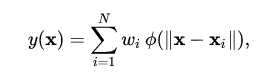### Subscribe to Our Bi-Weekly AI Newsletter

A radial basis function (RBF) is a function that assigns a real value to each input from its domain (it is a real-value function), and the value produced by the RBF is always an absolute value; i.e. it is a measure of distance and cannot be negative.

`f(x) = f(||x||)`

Euclidean distance, the straight-line distance between two points in Euclidean space, is typically used.

Radial basis functions are used to approximate functions, much as neural networks act as function approximators. The following sum:represents a radial basis function network. The radial basis functions act as activation functions.

The approximant `f(x)` is differentiable with respect to the weights `W`, which are learned using iterative updater methods commong among neural networks.